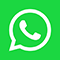HYBRID EVENT: You can participate in person at Tokyo, Japan or Virtually from your home or work.Azerbaijan National Academy of Science, Azerbaijan

#### Abstract:

Paper presents the results of modeling the optimal regime of the electrodeposition process of the antimony-selenium system Sb–Se. Based on the experimental data, a regression model was built using the BoxBenkinD module of the Matlab-7 software. The regression equation Y = f(X1,X2,X3,X4) establishes the functional dependence of the product yield Y ( in the form of deposition of the studied thin films of the Sb–Se antimony-selenium system) on 4 process parameters, where X1 is the concentration of SbOCl in the range of 0.01–0.09 mol/l; X2 - concentration of H2SeO3 (0.01–0.09 mol/l); X3 - current density (20–60 mA/cm2); X4 - temperature (25–850?). To solve the problem of process optimization, a specially developed by author software module was used. Restrictions are imposed on the objective function: 85% ≤ Y ≤ 100%. To search for the optimal region, the parameters of one of the local maxima were chosen from the experimental data as the starting point. Start point coordinates: X1=0.09, X2=0.01, X3=20 and X4=25, Ymax=87.5%.

In order to guarantee that the model provides high-precision predictions the regression analysis was implemented with the verification of the adequacy of the model (according to the Fisher criterion), as well as the study of the relative approximation error. The actual value of the Fisher distribution F-test: F = 89.07. Table value Ft (4;11) = 3.36. Since the actual value of F > Ft, and the average approximation error ? = 1.11%, and thus regression characteristics are statistically significant it was  concluded that the regression equation adequately describes this process.

Based on the constructed model, the response surface of the 2-parameter function Y = f(X1,X3) was built, with fixed values ??of the parameters X2 = 0.01, X4 = 25 at the local optimum point and given intervals for X1 , X3. A specially developed software module is implemented in the Excel environment using Microsoft Excel add-in programs -VBA, Solver for optimization in what-if analysis. The module uses procedures to regulate, redirect of variable’ cells content, which allows to conduct the calculations in interactive mode and get the distribution of function values ?? at the response surface on an Excel sheet. In the set up stage conditions are introduced in the calculation mode to highlight the cells of the values ??of the objective function with a certain fixed color in the optimal area. Thus, the function calculation area filled with a pre-selected color visually identifies the optimal modes of the process.

As a result of applying the module under a given condition 85% ≤ Y ≤ 98% for the process, an optimal area was obtained with the following boundaries: Ymax ? (85% ÷ 97,9%) at X1 ? (0.065 ÷ 0.089), X3 ? (20÷ 29) , at X2 = 0.01, X4 = 25. For more precision conditions for the optimal yield of the product 92% ≤ Y ≤ 98%, it was obtained: Ymax ? (92.4% ÷ 97.9%) at X1 ? (0.078 ÷ 0.089), X3 ? (20 ÷ 23.5) , at X2 = 0.01, X4 = 25.

Audience take-Away:

• Methods of identification of optimal regimes of chemical-technological processes
• Benefits of mathematical modeling for chemical-technological processes description
• Regression analysis application for verification of the adequacy of the model

The advantages of mathematical modeling of a chemical process can be divided into economic-environmental and cognitive benefits. The main economic and ecological aspects of the advantages of modeling: reduced time spent on research; minimizing the use of consumables, reagents, toxic chemicals, devices used during the experiments; research costs are reduced. The benefits of a cognitive nature are associated with obtaining new information, knowledge about the process. An important function of the model is to identify the complexity of the process, to clarify the structure of the process or object under study, to streamline, highlight and identify new connections in the process under study. As a result, new ideas and a practical result should be proposed based on the model.

#### Biography:

Ch. M. Shabanova studied Mathematics at the Baku State University, Azerbaijan and graduated as MS in 1982. She in 1984 joined the research group of Prof. R.M.  Kasimov at the Institute of Catalysis and Inorganic Chemistry, Azerbaijan National Academy of Sciences (ICIC-ANAS) as senior researcher since 2004 and in 2016 joined the research group of Prof. M. Asadov at the same institution. She has published more than 40 research articles and theses. The main directions of scientific investigations: visual modeling of optimal areas for chemical-technological processes; visual mapping of phase diagrams of the multicomponent systems;  polar liquids identification  by construction of the polar liquid response surface using  remote sensing method, development of pilot register of release and transfer of pollution (PRTR) from stationary sources in order to investigate the environmental pollution by toxic chemicals.